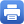Columbia Home
April 19: Jean Bertoin (Zurich)

Title: A probabilistic approach to spectral analysis of growth-fragmentation equations

Abstract:

(based on a joint work with Alex Watson, Manchester University).

The growth-fragmentation equation describes a system of growing and dividing particles, and arises in models of cell division, protein polymerisation and even telecommunications protocols. Several important questions about the equation concern the asymptotic behaviour of solutions at large times: at what rate do they converge to zero or infinity, and what does the asymptotic profile of the solutions look like? Does the rescaled solution converge to its asymptotic profile at an exponential speed? These questions have traditionally been studied using analytic techniques such as entropy methods or splitting of operators. In this work, we present a probabilistic approach to the study of this asymptotic behaviour. We use a Feynman–Kac formula to relate the solution of the growth-fragmentation equation to the semigroup of a Markov process, and characterise the rate of decay or growth in terms of this process. We then identify the Malthus exponent and the asymptotic profile in terms of a related Markov process, and give a spectral interpretation in terms of the growth-fragmentation operator and its dual.

Wednesday, April 19, 4:30 – 5:30 p.m.

Mathematics 520
Tea will be served at 4:00 p.m.Print this page